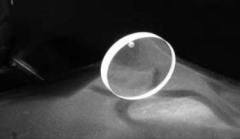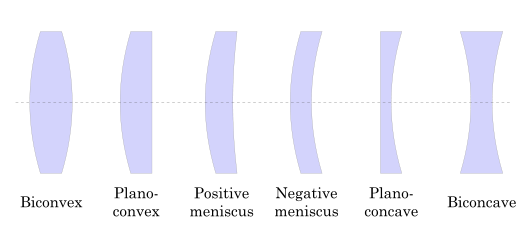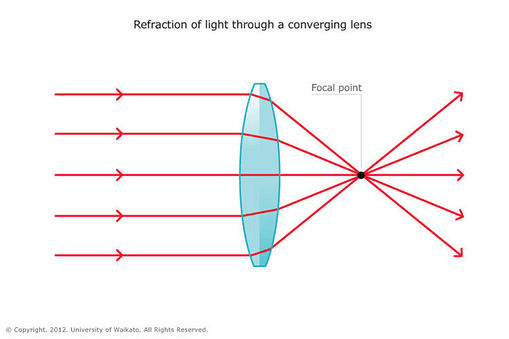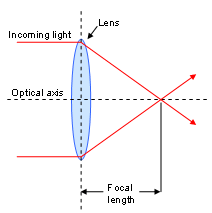## لنز اپتیکی

شرکت نور ایمن تجهیز تامین کننده لنز های اپتیکی و UV می باشد . این لنزها مناسب برای محدوده فرابفنش، مرئی و مادون قرمز است. لنزهای UV از جنس Fused silica ساخته می شوند که بر خلاف لنزهای معمولی نور UV را جذب نمی کنند. این لنزها در ابعاد و فواصل کانونی مختلف و متناسب با نوع آزمایش ساخته می شوند. از جمله کاربردهای لنز اپتیکی می توان به تنظیم تمرکز نور در محل مورد نظر از نمونه تحت آزمایش اشاره نمود. همچنین در برخی آزمایش ها نظیر: پراکندگی رامان برای جمع آوری سیگنال های رامان و هدایت به سمت سیستم طیف سنجی از لنز استفاده می شود.## Optical Lenses InformationAn optical lens is a transparent optical component used to converge or diverge light emitted from a peripheral object. The transmitted light rays then form a real or virtual image of the object.

Lenses are a good example of transmissive optical components, meaning that they pass or transmit light. Other transmissive components include filterswindowsflatsprismsbeamsplitters, and waveplates, while the opposite category — reflectives (which reflect light rather than transmit it) — include optical mirrors and retroreflectors.

Optical lenses have been used since at least c. 700 BCE for a variety of applications, including:

• Magnification
• Correction of optical aberrations
• Use as a firestarter (burning-glasses)
• Image focusing
• Image projection

## Shapes and Types

Lenses come in a variety of shapes including biconvex, biconcave, plano-convex, plano-concave, positive meniscus and negative meniscus.Lens shapes. Image credit: Wikipedia

Lenses can be classified into two broad types: positive (or converging) and negative (or diverging) lenses.

Positive lenses cause a collimated beam of light — assuming the beam travels parallel to the lens axis and passes through the lens — to converge or focus on a spot behind the lens. When referring to the image above, biconvex and plano-convex lenses are considered positive.Refraction of light through a converging lens. Image credit: Science Learning Hub

Negative lenses cause a collimated light beam to diverge and spread behind the lens. The two types of concave lenses — biconcave and plano-concave — are negative.Refraction through a diverging lens. Image credit: Science Learning Hub

Meniscus lenses — a third broad type also referred to as convex-concave — can be either positive or negative, depending on the curvature of both sides of the lens. A meniscus lens with a steep concave surface is negative, while a lens with a steeper convex surface will be positive. A meniscus lens with equal curvature on both sides would neither converge nor diverge light.

Selecting the correct lens type and polarity is heavily dependent upon the intended application, as described below. Choosing an ideal lens shape — known as the application’s “best form” — is key to minimizing optical distortion and aberration.

## Focal Length, Conjugate Ratio, and Lens Selection

A lens’s focal point is the point on the optical axis where light converges. Its focal length is the distance from the lens to this point, as indicated in the image. A positive lens has a positive focal length, while a negative lens has a focal length less than zero. The image below illustrates these two parameters.Image credit: Australian Customs and Border Protection

The conjugate ratio is defined as the ratio between the distance from the object (light source) to the lens and the distance from the lens to the projected image. The endpoints of these two lengths are known as the object and image points. These two points lie on the lens’s optical axis and are positioned so that light emitted from the object point will be focused at the image point. An object placed at the focal point of a lens results in an infinite conjugate ratio, while an object placed at twice the focal length results in an image formed at twice the focal length, giving a conjugate ratio of 1.

The images below illustrate the important optical points.Image credit: Olympus

An application’s conjugate ratio largely determines the ideal type of spherical lens. The table below shows the ideal lens shapes for an application’s conjugate ratio.